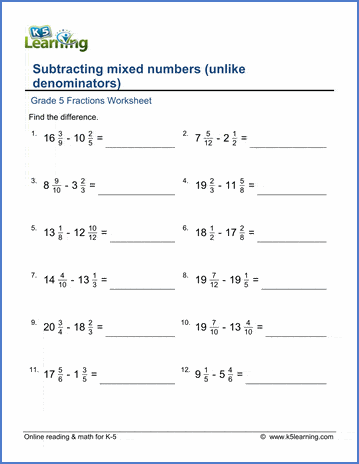# Subtract mixed numbers (unlike denominators)

## Fractions worksheets: Subtracting mixed numbers with unlike denominators

Below are six versions of our grade 5 math worksheet on subtracting mixed numbers from mixed numbers where the fractional parts have different denominators. These worksheets are pdf files.## More fractions worksheets

Explore all of our fractions worksheets, from dividing shapes into "equal parts" to multiplying and dividing improper fractions and mixed numbers.

## What is K5?

K5 Learning offers reading and math worksheets, workbooks and an online reading and math program for kids in kindergarten to grade 5.  We help your children build good study habits and excel in school.